# Bayesian Estimation of Generalized Graded Unfolding Model Parameters

The Generalized Graded Unfolding Model (GGUM) (Roberts, Donoghue, and Laughlin 2000) is an item response model designed to allow for disagreement from both ends of the latent space. bggum provides R tools for Bayesian estimation of GGUM parameters. This vignette provides a brief introduction to the GGUM and an overview of our posterior sampling algorithm before going through a simple example of preparing data, sampling, and analyzing the posterior samples. The vignette provides a practical guide; for more details and theoretical discussion of the GGUM, please see Roberts, Donoghue, and Laughlin (2000) or Duck-Mayr and Montgomery (2019). Duck-Mayr and Montgomery (2019) also provides more detail behind the development of our sampling algorithm; some of the more technical parts of this vignette closely follow the presentation there.

## The GGUM

We use the GGUM to study respondents’ responses to categorical items. The number of respondents is denoted by $$n$$, and respondents are indexed by $$i$$. The number of items is denoted by $$m$$, and items are indexed by $$j$$. Each item $$j$$ has observable response categories $$\{0, \ldots, K_j - 1\}$$; that is, $$K_j$$ indicates the number of response options for item $$j$$, and the response options are zero-indexed.

Following convention, we denote the probability of $$i$$ choosing option $$k$$ for item $$j$$ as $$P(y_{ij}=k|\theta_i) = P_{jk}(\theta_i)$$. Then

$\begin{equation} \label{eq:ggum-response-probability} P_{jk}(\theta_i) = \frac{\exp (\alpha_j [k (\theta_i - \delta_j) - \sum_{m=0}^k \tau_{jm}]) + \exp (\alpha_j [(2K - k - 1) (\theta_i - \delta_j) - \sum_{m=0}^k \tau_{jm}])}{\sum_{l=0}^{K-1} [\exp (\alpha_j [l (\theta_i - \delta_j) - \sum_{m=0}^l \tau_{jm}]) + \exp (\alpha_j [(2K - l - 1) (\theta_i - \delta_j) - \sum_{m=0}^l \tau_{jm}])]}, \end{equation}$

where

• $$\alpha_j$$ is the discrimination parameter for item $$j$$,
• $$\delta_j$$ is the location parameter for item $$j$$,
• $$\theta_i$$ is respondent $$i$$’s latent trait, and
• $$\tau_j$$ is the vector of option threshold parameters for item $$j$$, with $$\tau_{j0} \triangleq 0$$.

## Estimating GGUM Parameters

Roberts, Donoghue, and Laughlin (2000), the original paper developing the GGUM, provides an estimation procedure where item parameters are estimated using a marginal maximum likelihood approach and the $$\theta$$ parameters are then calculated by an expected a posteriori estimator. de la Torre, Stark, and Chernyshenko (2006) provides a Bayesian approach to estimation via Markov chain Monte Carlo (MCMC).

However, for the reasons discussed in Duck-Mayr and Montgomery (2019), we prefer a Metropolis coupled Markov chain Monte Carlo (MC3) approach (Gill 2008, 512–13; Geyer 1991). In MC3 sampling, we use $$N$$ parallel chains at inverse “temperatures” $$\beta_1 = 1 > \beta_2 > \ldots > \beta_N > 0$$. We update parameters for each chain using Metropolis-Hastings steps. The “temperatures” modify the probability of accepting proposals; the probability $$p$$ of accepting a proposed parameter value becomes $$p^{\beta_b}$$, so that chains become increasingly likely to accept all proposals as $$\beta \rightarrow 0$$. We then allow adjacent chains to “swap” states periodically as a Metropolis update. Only draws from the first “cold” chain are recorded for inference. (Interested readers can refer to Gill (2008) or Geyer (1991) for more details).

We follow de la Torre, Stark, and Chernyshenko (2006) in using the following priors:

\begin{align*} P(\theta_i) & \sim \mathcal{N}(0, 1), \\ P(\alpha_j) & \sim Beta(\nu_\alpha, \omega_\alpha, a_\alpha, b_\alpha), \\ P(\delta_j) & \sim Beta(\nu_\delta, \omega_\delta, a_\delta, b_\delta), \\ P(\tau_{jk}) & \sim Beta(\nu_\tau, \omega_\tau, a_\tau, b_\tau), \end{align*}

where $$Beta(\nu, \omega, a, b)$$ is the four parameter Beta distribution with shape parameters $$\nu$$ and $$\omega$$, with limits $$a$$ and $$b$$ (rather than $$0$$ and $$1$$ as under the two parameter Beta distribution). We then use the following MC3 algorithm to draw posterior samples:

• At iteration $$t = 0$$, set initial parameter values; by default we draw initial values from the parameters’ prior distributions.
• For each iteration $$t = 1, 2, \ldots, T$$:
• For each chain $$b = 1, 2, \ldots, N$$:
• Draw each $$\theta_{bi}^*$$ from $$\mathcal{N}\left(\theta_{bi}^{t-1}, \sigma_{\theta_i}^2\right)$$, and set $$\theta_{bi}^t = \theta_{bi}^*$$ with probability $$p\left(\theta_{bi}^*, \theta_{bi}^{t-1}\right) = \min\left\{1, \left(\frac{P\left(\theta_{bi}^*\right) L\left(X_i | \theta_{bi}^*, \alpha_{b}^{t-1}, \delta_{b}^{t-1}, \tau_{b}^{t-1}\right)}{P\left(\theta_{bi}^{t-1}\right) L\left(X_i | \theta_{bi}^{t-1}, \alpha_b^{t-1}, \delta_b^{t-1}, \tau_b^{t-1}\right)}\right)^{\beta_b}\right\}$$; otherwise set $$\theta_{bi}^t = \theta_{bi}^{t-1}$$.
• Draw each $$\alpha_{bj}^*$$ from $$\mathcal{N}\left(\alpha_{bj}^{t-1}, \sigma_{\alpha_j}^2\right)$$, and set $$\alpha_{bj}^t = \alpha_{bj}^*$$ with probability $$p\left(\alpha_{bj}^*, \alpha_{bj}^{t-1}\right) = \min\left\{1, \left(\frac{P\left(\alpha_{bj}^*\right) L\left(X_j | \theta_{b}^{t}, \alpha_{bj}^*, \delta_{bj}^{t-1}, \tau_{bj}^{t-1}\right)}{P\left(\alpha_{bj}^{t-1}\right) L\left(X_j | \theta_b^{t}, \alpha_{bj}^{t-1}, \delta_{bj}^{t-1}, \tau_{bj}^{t-1}\right)}\right)^{\beta_b}\right\}$$; otherwise set $$\alpha_{bj}^t = \alpha_{bj}^{t-1}$$.
• Draw each $$\delta_{bj}^*$$ from $$\mathcal{N}\left(\delta_{bj}^{t-1}, \sigma_{\delta_j}^2\right)$$, and set $$\delta_{bj}^t = \delta_{bj}^*$$ with probability $$p\left(\delta_{bj}^*, \delta_{bj}^{t-1}\right) = \min\left\{1, \left(\frac{P\left(\delta_{bj}^*\right) L\left(X_j | \theta_b^{t}, \alpha_{bj}^{t}, \delta_{bj}^*, \tau_{bj}^{t-1}\right)}{P\left(\delta_{bj}^{t-1}\right) L\left(X_j | \theta_b^{t}, \alpha_{bj}^{t}, \delta_{bj}^{t-1}, \tau_{bj}^{t-1}\right)}\right)^{\beta_b}\right\}$$; otherwise set $$\delta_{bj}^t = \delta_{bj}^{t-1}$$.
• Draw each $$\tau_{bjk}^*$$ from $$\mathcal{N}\left(\tau_{bjk}^{t-1}, \sigma_{\tau_j}^2\right)$$, and set $$\tau_{bjk}^t = \tau_{bjk}^*$$ with probability $$p\left(\tau_{bjk}^*, \tau_{bjk}^{t-1}\right) = \min\left\{1, \left(\frac{P\left(\tau_{bjk}^*\right) L\left(X_j | \theta_b^{t}, \alpha_{bj}^t, \delta_{bj}^t, \tau_{bj}^*\right)}{P\left(\tau_{bjk}^{t-1}\right) L\left(X_j | \theta_b^t, \alpha_{bj}^{t}, \delta_{bj}^{t}, \tau_{bj}^{t-1}\right)}\right)^{\beta_b}\right\}$$; otherwise set $$\tau_{bjk}^t = \tau_{bjk}^{t-1}$$.
• For each chain $$b = 1, 2, \ldots, N-1$$: Swap states between chains $$b$$ and $$b+1$$ (i.e., set $$\theta_b^t = \theta_{b+1}^t$$ and $$\theta_{b+1}^t = \theta_b^t$$, etc.) via a Metropolis step; the swap is accepted with probability $\min\left\{1, \frac{P_b^{\beta_{b+1}} P_{b+1}^{\beta_b}}{P_{b+1}^{\beta_{b+1}} P_{b}^{\beta_b}}\right\},$ where $$P_b = P(\theta_b)P(\alpha_b)P(\delta_b) P(\tau_b) L(X|\theta_b, \alpha_b, \delta_b, \tau_b)$$.

## Example

The high-level how-to for going from data to estimates follows six steps:

1. Correctly structure the data so that it is an integer matrix containing only responses, with respondents as rows and items as columns.
2. (Optional, but encouraged) Tune the standard deviations for proposal densities (using tune_proposals()) and the temperature schedule for the parallel chains (using tune_temperatures()).
3. Sample the posterior, preferably including a “burn-in” period (using ggumMC3()).
4. Post-process the output to identify which of the model’s reflective modes should be summarized (using post_process()).
5. Assess convergence (we use tools from the package coda for this purpose) and censoring (discussed below).
6. Obtain estimates using summary(). You can then view the items’ response functions and characteristic curves using irf() and icc().

To illustrate how to use the package, we will use data on justices’ votes in cases at the U.S. Supreme Court. The Supreme Court Database (Spaeth et al. 2019) provides data on all cases decided by the Supreme Court, including the individual justices’ votes. We will use a subset of this dataset giving the justices’ votes in cases decided during the Roberts Court (that is, since John Roberts because the Chief Justice of the United States).1

### 1. Prepare the data

This data is arranged so that each row records a justice’s vote in a case:

roberts_court <- read.csv("roberts_court.csv", stringsAsFactors = FALSE)
head(roberts_court)
#>     caseId            lexisCite term justiceName vote
#> 1 2005-005 2005 U.S. LEXIS 8373 2005   JPStevens    1
#> 2 2005-005 2005 U.S. LEXIS 8373 2005   SDOConnor    1
#> 3 2005-005 2005 U.S. LEXIS 8373 2005     AScalia    1
#> 4 2005-005 2005 U.S. LEXIS 8373 2005   AMKennedy    1
#> 5 2005-005 2005 U.S. LEXIS 8373 2005    DHSouter    1
#> 6 2005-005 2005 U.S. LEXIS 8373 2005     CThomas    1

We need to do three things with this data:

• Recode the responses (the variable vote) to be integers in $$\{0, \ldots, K_j - 1\}$$. In this case we will be using a dichotomous coding where $$1$$ is voting for the same outcome as the majority coalition and $$0$$ is voting for the opposite outcome.
• Reshape the data so that a row is a justice’s response to every case rather than a row being a justice’s response to one case.
• Eliminate all unanimous votes

Here’s one way to accomplish those goals:

## Recode the votes to be integers in $\{0, \ldots, K_j - 1\}$
roberts_court$vote <- ifelse(roberts_court$vote %in% c(2, 7), 0, 1)
## Reshape the data
library(dplyr)
#>
#> Attaching package: 'dplyr'
#> The following objects are masked from 'package:stats':
#>
#>     filter, lag
#> The following objects are masked from 'package:base':
#>
#>     intersect, setdiff, setequal, union
library(tidyr)
responses <- roberts_court %>%
select(-caseId, -term) %>%
spread(lexisCite, vote)
## I like to keep the respondents' names as the rownames
rownames(responses) <- responses$justiceName ## Now we just eliminate the respondent ID column and turn it into a matrix responses <- as.matrix(responses[ , -1]) ## Eliminate unanimous items unanimous <- apply(responses, 2, function(x) length(unique(na.omit(x))) == 1) responses <- responses[ , !unanimous] ## Here are responses to a few items: responses[ , c(1, floor(ncol(responses) / 2), ncol(responses))] #> 2005 U.S. LEXIS 8554 2012 U.S. LEXIS 2712 2019 U.S. LEXIS 725 #> AMKennedy 1 1 NA #> AScalia 1 1 NA #> BMKavanaugh NA NA 1 #> CThomas 1 1 1 #> DHSouter 1 NA NA #> EKagan NA 0 0 #> JGRoberts 1 1 0 #> JPStevens 1 NA NA #> NMGorsuch NA NA 1 #> RBGinsburg 0 0 0 #> SAAlito NA 1 1 #> SDOConnor 1 NA NA #> SGBreyer 0 0 1 #> SSotomayor NA 0 0 ### 2. Tune Hyperparameters You can use any positive value for the proposal densities’ standard deviations, and any value in $$(0, 1]$$ for the inverse “temperatures,” but we provide routines to generate hyperparameters to help you more efficiently sample the posterior. To tune the proposals, we start with proposal standard deviations of $$1$$, then run iterations of the sampler, periodically incrementing or decrementing the standard deviations to reach an optimal rejection rate. To tune the temperatures, we implement the optimal temperature finding algorithm from Atchadé, Roberts, and Rosenthal (2011). Here’s how we’d do that in this case ## Load the package library(bggum) ## Set the seed for reproducibility set.seed(123) ## Tune the proposal densities proposal_sds <- tune_proposals(responses, tune_iterations = 5000) set.seed(456) ## Tune the temperature schedule temps <- tune_temperatures(responses, n_temps = 6, proposal_sds = proposal_sds) The temperature finding routine also relies on running iterations of the sampler (though the tuning process is quite different). Although in the authors’ experience it happens very rarely, with finite iterations it is possible for the temperature schedule tuning algorithm to become stuck in a non-optimal region resulting in a decidedly not optimal temperature schedule. We recommend examining the temperature schedule before proceeding to ensure this has not occurred: round(temps, 2) #>  1.00 0.93 0.87 0.81 0.76 0.71 The most sure sign of a problem is a very large jump (e.g. going from 1 as the first inverse temperature to 0.1 as the second) or a very small jump (e.g. going from 1 to 0.999). Here, as is typical, there were no issues. ### 3. Sample the posterior We suggest running multiple chains to be able to assess convergence, and to run those chains in parallel: ## We need the parallel package library(parallel) ## We'll set up the cluster with two cores (for two chains) cl <- makeCluster(2, type = "FORK", outfile = "bggum-log.txt") ## Deal with reproducibility clusterSetRNGStream(cl = cl, iseed = 789) ## Produce the chains chains <- parLapplyLB(cl = cl, X = 1:2, fun = function(x) { ggumMC3(data = responses, sample_iterations = 50000, burn_iterations = 5000, proposal_sds = proposal_sds, temps = temps) }) ## Stop the cluster stopCluster(cl) ### 4. Post process The GGUM is subject to reflective invariance; the likelihood of a set of responses given $$\theta$$ and $$\delta$$ vectors is equal to the the likelihood given vectors $$-\theta$$ and $$-\delta$$. While sometimes informative priors on a few respondents or items is used to identify models with rotational invariance during sampling, we find it more reliable to handle identification in post-processing (Duck-Mayr and Montgomery 2019). (For the validity of this approach to solving reflective invariance, see Proposition 3.1 and Corollary 3.2 in (Stephens 1997)). In this example, the reflective modes are one in which all the liberal justices, such as Justice Ruth Bader Ginsburg, are scored positively on the latent scale and all the conservative justices, such as Justice Clarence Thomas, are scored negatively; and one in which all the liberal justices are scored negatively on the latent scale and all the conservative justices are scored positively. We will use Justice Ginsburg as a constraint to post-process our posterior samples: constraint <- which(rownames(responses) == "RBGinsburg") processed_chains <- lapply(chains, post_process, constraint = constraint, expected_sign = "-") The key is to select a respondent or item for which you have good reason to believe that their posterior density should not have appreciable mass on the “wrong side” of $$0$$. We can check how well this worked by looking at the posterior samples for other justices; here, for example, are the raw posterior samples (which freely sample from both reflective modes) and the post-processed samples for Chief Justice John Roberts, a reliable (though not extreme) conservative: roberts <- which(rownames(responses) == "JGRoberts") trace_colors <- c("#0072b280", "#d55e0080") iters <- nrow(chains[]) idx <- seq(floor(iters / 1000), iters, floor(iters / 1000)) chain1_raw <- chains[][idx, roberts] chain2_raw <- chains[][idx, roberts] chain1_pp <- processed_chains[][idx, roberts] chain2_pp <- processed_chains[][idx, roberts] ylims <- range(c(chain1_raw, chain2_raw, chain1_pp, chain2_pp)) opar <- par(mar = c(3, 3, 3, 1) + 0.1) plot(idx, chain1_raw, type = "l", col = trace_colors, ylim = ylims, xlab = "", ylab = "", xaxt = "n", yaxt = "n", main = "Raw Samples") lines(idx, chain2_raw, col = trace_colors) axis(side = 1, tick = FALSE, line = -0.75) axis(side = 2, tick = FALSE, line = -0.75) title(xlab = "Iteration", ylab = expression(theta[Roberts]), line = 1.5)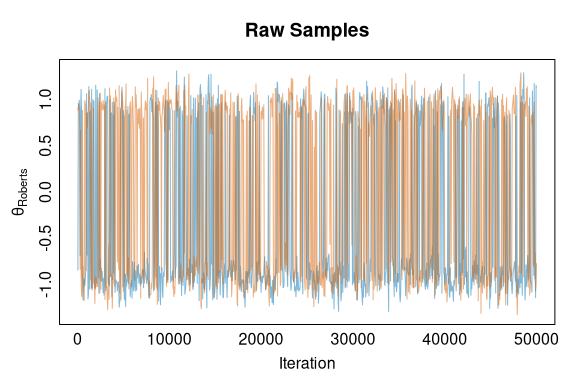plot(idx, chain1_pp, type = "l", col = trace_colors, ylim = ylims, xlab = "", ylab = "", xaxt = "n", yaxt = "n", main = "Processed Samples") lines(idx, chain2_pp, col = trace_colors) axis(side = 1, tick = FALSE, line = -0.75) axis(side = 2, tick = FALSE, line = -0.75) title(xlab = "Iteration", ylab = expression(theta[Roberts]), line = 1.5)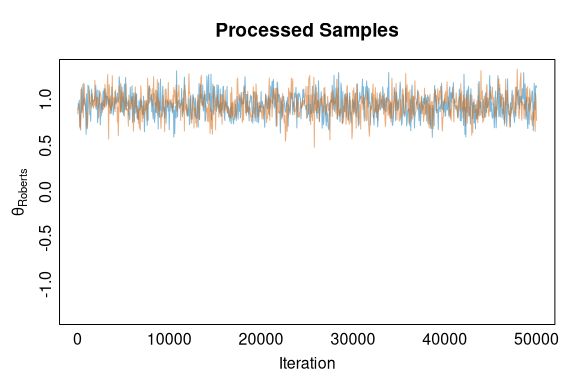par(opar) ### 5. Assess convergence As with any Bayesian model, it is important to assess convergence. ggumMC3() returns an object that has as one of its classes mcmc (with the necessary relevant attributes) so that coda tools can be used for this purpose: library(coda) ## Look at the Gelman-Rubin potential scale reduction factor: convergence_stats <- gelman.diag(processed_chains) ## See what estimates are: summary(convergence_stats$psrf[ , 1])
#>    Min. 1st Qu.  Median    Mean 3rd Qu.    Max.
#>   1.000   1.000   1.000   1.001   1.001   1.008

One additional issue that must be tended to before confidently proceeding to the estimates is censoring. Recall the item parameter priors censor allowed draws to be within $$[a, b]$$. You must take care that the prior hyperparameters are chosen so they do not bias the posterior via censoring. In practice, the default limits on $$\delta$$ ($$a = -5, b = 5$$) and $$\tau$$ ($$a = -6, b = 6$$) have not presented the authors with any issues. Likewise, the default limits on $$\alpha$$ ($$a = 0.25, b = 4$$) do not usually present issues; however, the authors have observed censoring when analyzing rollcall data from the U.S. Congress, which was fixed by expanding the limits. In this U.S. Supreme Court application, no censoring was observed.

### 6. Obtain estimates

Now that we have sampled the posterior, post-processed the output, and assessed convergence, obtaining respondent and item parameters is easily done by calling summary():

posterior_summary <- summary(processed_chains)

The summary will be a list of three elements: the parameter estimates (posterior means), the posterior standard deviations, and a matrix of summary statistics of the posterior draws—the mean (estimates), median, standard deviations, and 0.025 and 0.975 quantiles:

str(posterior_summary$estimates, max.level = 1) #> List of 4 #>$ theta: Named num [1:14] 0.281 1.655 0.704 2.01 -1.822 ...
#>   ..- attr(*, "names")= chr [1:14] "theta1" "theta2" "theta3" "theta4" ...
#>  $alpha: Named num [1:556] 1.566 0.843 2.209 2.215 1.997 ... #> ..- attr(*, "names")= chr [1:556] "alpha1" "alpha2" "alpha3" "alpha4" ... #>$ delta: Named num [1:556] 1.336 0.444 -1.321 -1.348 -2.081 ...
#>   ..- attr(*, "names")= chr [1:556] "delta1" "delta2" "delta3" "delta4" ...
#>  $tau :List of 556 str(posterior_summary$sds, max.level = 1)
#> List of 4
#>  $theta_sds: Named num [1:14] 0.133 0.145 0.27 0.136 0.181 ... #> ..- attr(*, "names")= chr [1:14] "theta1" "theta2" "theta3" "theta4" ... #>$ alpha_sds: Named num [1:556] 0.809 0.458 0.905 0.901 0.875 ...
#>   ..- attr(*, "names")= chr [1:556] "alpha1" "alpha2" "alpha3" "alpha4" ...
#>  $delta_sds: Named num [1:556] 1.045 1.542 0.947 0.945 0.934 ... #> ..- attr(*, "names")= chr [1:556] "delta1" "delta2" "delta3" "delta4" ... #>$ tau_sds  :List of 556
head(posterior_summary$statistics) #> Quantile 0.025 Median Mean Quantile 0.975 Posterior SD #> theta1 0.02001687 0.2805174 0.2810517 0.5388162 0.1330324 #> theta2 1.36899880 1.6560695 1.6548188 1.9364069 0.1451344 #> theta3 0.17241403 0.7054925 0.7041570 1.2307585 0.2703325 #> theta4 1.74518893 2.0086999 2.0098053 2.2788369 0.1359351 #> theta5 -2.17762468 -1.8206694 -1.8215464 -1.4656411 0.1806853 #> theta6 -1.58472032 -1.2929888 -1.2934368 -1.0032852 0.1472650 Let’s see how the justices’ rank ideologically according to the GGUM: theta <- posterior_summary$estimates$theta names(theta) <- rownames(responses) n <- length(theta) ordering <- order(theta) theta_sorted <- sort(theta) opar <- par(mar = c(3, 6, 1, 1) + 0.1) plot(theta_sorted, factor(names(theta_sorted), levels = names(theta_sorted)), pch = 19, xlim = c(-3, 2.5), xaxt = "n", yaxt = "n", xlab = "", ylab = "") axis(side = 1, tick = FALSE, line = -0.75) axis(side = 2, tick = FALSE, line = -0.75, las = 1, labels = names(theta_sorted), at = 1:n) title(xlab = expression(theta), line = 1.5) segments(x0 = posterior_summary$statistics[ordering, 1], y0 = 1:n,
x1 = posterior_summary$statistics[ordering, 4], y1 = 1:n)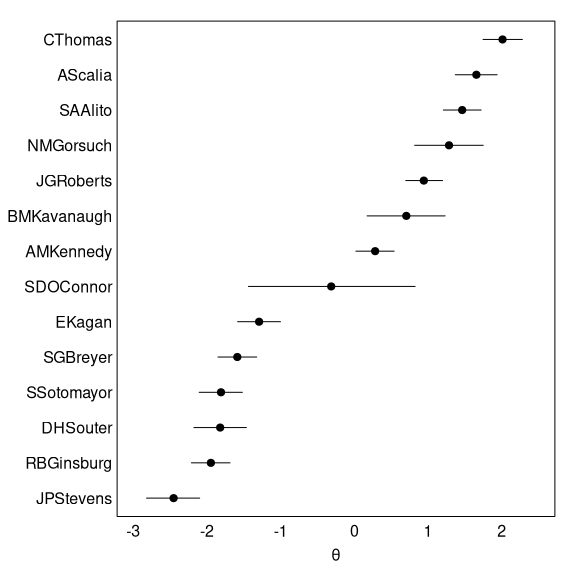par(opar) The justices are arrayed on the left-right continuum largely as we’d expect (Justices John Paul Stevens, Ruth Bader Ginsburg, and Sonia Sotomayor well to the left; Justices Clarence Thomas, Antonin Scalia, and Samuel Alito well to right; Justice Anthony Kennedy just right of center). The estimates are also fairly precise, with the exception of Justice Sandra Day O’Connor (who only participated in 1.5% of the cases in our data), Justice Brett Kavanaugh (who only participated in 6.1% of the cases in our data), and Justice Neil Gorsuch (who participated in 14.5% of the cases in our data). We can also observe the item characteristic curves: alpha <- posterior_summary$estimates$alpha delta <- posterior_summary$estimates$delta tau <- posterior_summary$estimates\$tau
phillip_morris <- which(colnames(responses) == "2007 U.S. LEXIS 1332")
rucho <- which(colnames(responses) == "2019 U.S. LEXIS 4401")
icc(alpha[phillip_morris], delta[phillip_morris], tau[[phillip_morris]],
main_title = "Philip Morris USA, Inc. v. Williams",
plot_responses = TRUE, thetas = theta,
responses = responses[ , phillip_morris])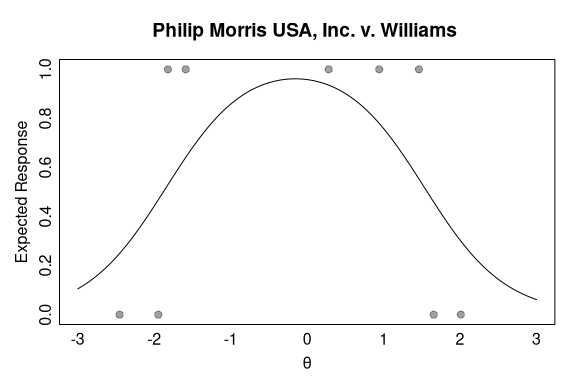icc(alpha[rucho], delta[rucho], tau[[rucho]],
main_title = "Rucho v. Common Cause",
plot_responses = TRUE, thetas = theta,
responses = responses[ , rucho])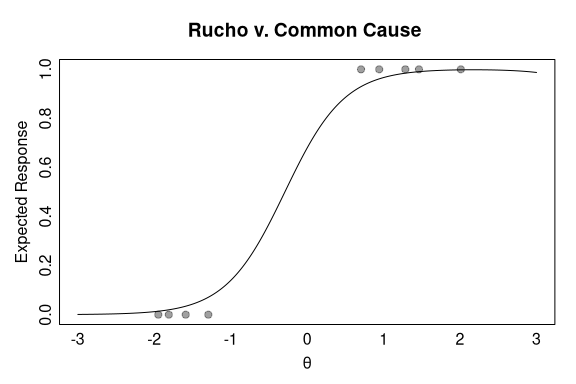(Item response functions are also available via irf()).

1. We have excluded from this dataset cases decided with no oral argument and equally divided cases. The code to reproduce all data used in this vignette can be found in the data-raw/ sub-directory of our GitHub repository (https://github.com/duckmayr/bggum).↩︎